# University of Virginia Library

Search this document

### 5. A complementary approach to evaluation and history.

5.1. The main drawbacks of the above approach are the need to compare a large array of error percentages in each variant passage and the failure to suggest a textual history. The following complementary method aims to fill these gaps.

Suppose a two-dimensional map of manuscripts, on which the distances between points reflect the degrees of textual divergence between the corresponding manuscripts (cf. §3.2). To such a map we might add hypothetical manuscripts. Imagine, for example, a manuscript which always follows either A or B. We may expect to locate it along the line AB, in a position reflecting its relative indebtedness to its two components. Similarly, a hypothetical manuscript conflated from ABC should lie within the triangle ABC, and a mixture of many manuscripts should lie within the largest polygon that can be formed from the corresponding points (cf. fig. 19). These expectations are merely intuitive, and we shall see that they need modification; but for the moment let us accept them.

The best text recoverable from the extant manuscripts is a hypothetical mixture ω which selects the true reading wherever that reading survives, but follows the manuscripts where all err together. In an uncontaminated tradition, ω would be the archetype, which term denotes

107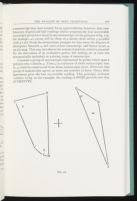a manuscript that once existed. In an open tradition, however, that combination of good and bad readings which comprises the best recoverable text might never have stood in any manuscript; in the pedigree of fig. 14a, for example, ω's errors will be those of a minus those which γ avoided with λ's aid. From the determinate passages we may assess the degrees of divergence between ω and each extant manuscript, and hence locate ω on the map. This step introduces the notion of priority, which is essential for the derivation of an evaluative policy, but lacking (or at least not systematically included) in existing maps of manuscripts.

Consider a group of manuscripts represented by points which span a polygon that contains ω. Then ω is a mixture of those manuscripts; that is, ω could be constructed from those manuscripts alone. Wherever that group of manuscripts agrees, so must any mixture of them. Hence their agreement gives the best recoverable reading. This principle evaluates variants: in fig. 20, for example, the reading of OPQX prevails over that of NRSTVYZ.

108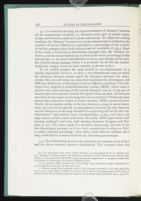5.2. To construct the map, we require measures of 'distance' between all the manuscripts involved, i.e. between every pair of extant manuscripts and between ω and each extant manuscript. To adjust for missing readings, the 'distance' between two extant manuscripts is defined as the number of textual differences, expressed as a percentage of the number of variant passages where both manuscripts are available (cf. §4.5). Since at the outset ω is known in determinate passages only, the 'distance' between ω and an extant manuscript is taken as the error percentage of that manuscript, i.e. its total of identifiable errors as a percentage of the number of determinate passages where it is available. In all but the smallest traditions, unique errors are best omitted, as shown in §5.7.

To be readily grasped the map should be two-dimensional. It is usually impossible, however, to draw a two-dimensional map on which the distances between points equal the distances between the manuscripts. One can only draw up a map that minimises the discrepancy, and different definitions of discrepancy lead to different methods. The technique here adopted is multidimensional scaling (MDS), which aims to preserve the rank ordering of the textual distances; that is, if one pair of manuscripts shows greater textual divergence than another, the first pair should be farther apart on the map than the second pair. Although most manuscript maps have rested on factor analysis, MDS is preferred here. Firstly, factor analysis yields, in the first instance, a map in many dimensions, and aims for no specific correspondence between the data distances and the distances on the map obtained by neglecting all but the first two dimensions; and without such correspondence, as §5.4 will show, the map cannot reliably assist evaluation. Secondly, MDS copes better with missing readings and even with missing distances (Lingoes and Roskam, p. 73). The latter result if a lacunose manuscript survives in too few determinate passages, or if two such manuscripts overlap too little, to yield a reliable percentage. That entry could then be omitted, and a map could still be constructed from the remaining percentages.

5.3. The relationship between the measures of common error in §4.2 and the above statistics deserves clarification. The variants where any

109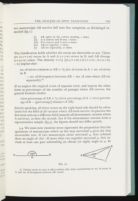two manuscripts AB survive fall into five categories, as Kleinlogel remarked (§3.1):
• (i) AB agree in the correct reading, s times.
• (ii) A is correct and B errs, t times
• (iii) B is correct and A errs, u times
• (iv) AB err together, v times
• (v) AB err separately, w times
The classification holds whether the errors are detectable or not. There are (u+v+w) errors in A and (t+v+w) errors in B, and AB diverge (t+u+w) times. The identity v=1/2 [(u+v+w)+(t+v+w)-(t+u+w) -w] implies that:

no. of errors common to AB = 1/2 (no. of errors in A + no. of errors in B

— no. of divergences between AB — no. of cases where AB err separately).

If we neglect the atypical event of separate error, and express the other items as percentages of the number of passages where AB survive, the general formula results:

error percentage of AB equals2dot 1/2 (error percentage of A + error percentage of B - [percentage] distance of AB).
Strictly speaking, all three terms on the right-hand side should be calculated over the field of all variants where AB both survive. In practice the first term rests on a different field (namely all determinate variants where A survives), as does the second; but if the determinate variants form a representative sample (§4.3), the figures should not differ greatly.

5.4. We must now examine more rigorously the proposition that the agreement of manuscripts which on the map surround ω gives the best recoverable text. If two manuscripts alone surround ω, they subtend there an angle of 180°. If more than two together surround ω, they include at least one pair subtending an obtuse (or right) angle at ω. In

110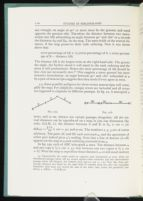any triangle, an angle of 90° or more must be the greatest and stand opposite the greatest side. Therefore the distance between two manuscripts (say AB) subtending an angle between 90° and 180° at ω exceeds the distances Aω and Bω, on the map. The same holds of the textual distances, if the map preserves their rank ordering. Now it was shown above that

error percentage of AB equals2dot 1/2 (error percentage of A + error percentage of B - distance AB)

The distance AB is the largest term on the right-hand side. The greater the angle, the further ahead it will stand in the rank ordering and the more it will predominate. Hence the error percentage of AB should be low—but not necessarily zero. This suggests a more general but more tentative formulation: an angle between 90° and 180° subtended at ω by a pair of manuscripts suggests that they rarely if ever agree in error.

5.5. Some possible pedigrees for three extant manuscripts will exemplify the map. For simplicity, unique errors are included and all errors are supposed to originate in different passages. In fig. 22, A miscopied ω

twice, and so on, whence ten variant passages altogether. All the textual distances can be reproduced on a map in just one dimension (fig. 22b): d(A,B), i.e. the distance between A and B, is 3/10 x 100 = 30; d(B,ω) = 3+2/10 x 100 = 50; and so on. The numbers 2, 3, 5 are of course arbitrary. The pairs AC and BC each surround ω, and the agreement of either pair indeed gives ω's reading. Note that a line of descent (A→B) appears on the map as a path radiating outwards from ω.

In fig. 23a, each of ABC miscopied ω once. The distance between ω and any copy is 1/3 x 100 ÷ 33; that between any two copies is 2/3 x 100 equalsdot 67. Were the map to reproduce those distances, ω would stand midway

111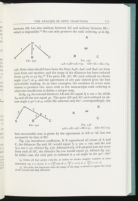between AB, but also midway between AC and midway between BC—which is impossible. We can only preserve the rank ordering, as in fig.23b. Here what should have been the lines AωB, AωC and BωC are bent away from one another, and the range of the distances has been reduced (from 33:67 to 37:63). The pairs AB, AC, BC each subtend an obtuse angle (120°) at ω, and the agreement of any pair indeed gives the best recoverable reading. As in later examples, the numbers of errors were chosen to produce ties, since with so few manuscripts rank ordering is otherwise insufficient to define a unique map.

In fig. 24, the textual distances AB and AC equal 4/5 x 100 = 80, while Aω and all the rest equal 40. The pairs AB and AC each subtend an obtuse angle (150°) at ω, while BC subtend only 60°; correspondingly, the

best recoverable text is given by the agreement of AB or AC but not necessarily by that of BC.

Fig. 25a introduces conflation. If B reproduced all errors of A and C, the distances Bω and AC would equal 4/4 x 100 = 100, and the rest 2/4 x 100 = 50, whence fig. 25b. Alternatively, if B adopted just one error from each of AC, the distance Bω too would equal 50, whence fig. 25c. In either case, the only pair to subtend at ω an angle in the 90°-180°

112range is AC (90° or 120°), while the remaining pairs subtend only 45° or 60°. The genealogy confirms that AB could agree in A's errors, and BC in C's, but that AC agree only if both reproduce ω. Note that the distance of a conflate manuscript from ω reflects its total of errors, while its direction thence is a compromise between those of its sources, reflecting their proportionate contributions.

5.6. The principle that paths radiating outwards from ω indicate descent, together with this remark on conflation, help to derive a textual history from the map, even though a unique history cannot be recovered (§§1.2, 2.1). We may indeed experiment with alternative histories, positing, for example, a lost common ancestor of two manuscripts (say AB) at the incentre of the triangle ABω. Thus the map of fig. 25c could be reinterpreted by supposing a lost common ancestor α for AB (ωαA and ωαB becoming somewhat bent (see n. 77) lines of descent) and regarding C as conflated from B and a lost source β (C's direction from ω lying between those of B and β). This pedigree (fig. 26b) wholly differs from fig. 25a, but explains equally well the survival of the truth now in A alone (untainted by B's errors), now in C alone (thanks to β), but never in B alone. External clues, such as dates, may help to choose between alternative histories.

5.7. One limitation of the method is that it may be impossible to draw any two-dimensional map that reflects the rank ordering adequately.

113The rank orderings of the textual and map distances are in fact unlikely to correspond exactly (unless the manuscripts are few), but the discrepancy is sometimes so extensive that the map is inapplicable. Suppose, for example, five independent copies of ω. No possible map can providethat every pair of manuscripts subtend an obtuse angle at ω. A compromise like fig. 27b, where some pairs subtend an obtuse angle and others do not, creates illusions, e.g. that B is conflated from AC. Such cases could be detected through the measures of 'badness of fit' output together with the map.

An implication of the last case is that unique errors should (rather than may—§4.2) be omitted here, in all but the smallest traditions. In most traditions at least five manuscripts bear unique errors. A map based on those errors alone would be at least as cramped and misleading as fig. 27b, and even a map based only in part on those passages might partially retain those defects.

The map is also limited in its capacity to convey complex relationships. Consider, for example, the addition to fig. 23b of a conflate manuscript drawn 40% from A, 40% from B, 20% from C. Its bearing from ω must lie between those of A and B, its main sources; but then it is

114indistinguishable from a mixture of AB alone. Again, in any tradition comprising three manuscripts ABC, such as those of fig. 22-25, suppose that we inserted for each manuscript five (say) instances where that manuscript was correct against the agreement of the others. Then the number of divergences between AB would rise by 5+5=10, as would the number of divergences between Aω, and similarly for all other pairs. The rank ordering, and therefore the map, would remain unchanged. However, any evaluative policy founded on the map would fall, since any manuscript even singly might now preserve the truth. Hence even when a map has straightforward implications for evaluation and history, the risk remains that some important relationship has been overlooked. A partial answer is that checks may exist. The former complication (regarding the manuscript conflated from ABC) might be traced through the entry for C and the conflate manuscript in the table of common error percentages (§4.5); the latter (each manuscript alone preserving some true readings) might be suspected from the incidence of unique preservation among determinate passages. If such danger signals are absent, however, and the map gives reasonable results, it seems over-cautious to posit further complications.

A problem in applying the map to evaluation is that the same error may arise by coincidence in two manuscripts which happen to subtend an obtuse angle at ω. A similar difficulty limits the stemma. Intrinsic considerations may indicate the likelihood of polygenesis of a given reading.

The map, then, must be used not in isolation but in conjunction with the error percentages. Where either method is indecisive, caution is necessary, as §6.3 illustrates.

In some respects the map is more limited than the stemma, but in other respects less so, whence the hope that the new methods may illuminate some traditions where the stemma fails. The virtue of the map is that of statistical procedures in general, namely the capacity to reveal fundamental trends, even though random fluctuations may disguise them, amid a mass of data. The genealogical relationships, however entangled, are fundamental in that they abide throughout the text. They are disguised by a host of sporadic (if not random) factors, e.g. coincidence, conjecture, physical damage and critical misjudgments among the supposedly determinate passages. The following experiment shows what the map can recover and apply to evaluation and history.